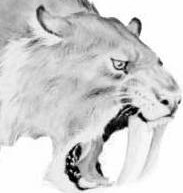# Sabre

SIGMA

### Sabre manual

Syntax: SIGMA number
or
SIGMA keyword=number [ keyword=number [ keyword=number]] where number is a positive real number and keyword is FIRST, SECOND or THIRD.

Purpose:
SIGMA number sets the initial value for the residual standard deviation in univariate linear (Gaussian) models.

SIGMA FIRST=number sets the initial value for the residual standard deviation in bivariate or trivariate models where the first response is linear.

SIGMA SECOND=number sets the initial value for the residual standard deviation in bivariate or trivariate models where the second response is linear.

SIGMA THIRD=number sets the initial value for the residual standard deviation in trivariate models where the third response is linear.

The current setting of the SIGMA parameter(s) may be obtained by issuing the DISPLAY SETTINGS command.

### Sabre manual

Go to: Sabre home page | Sabre manual | Downloading & Installing Sabre | Sabre examples | Training materials | Sabre mailing list | Contact us

Other links: Centre for e-Science | Centre for Applied Statistics